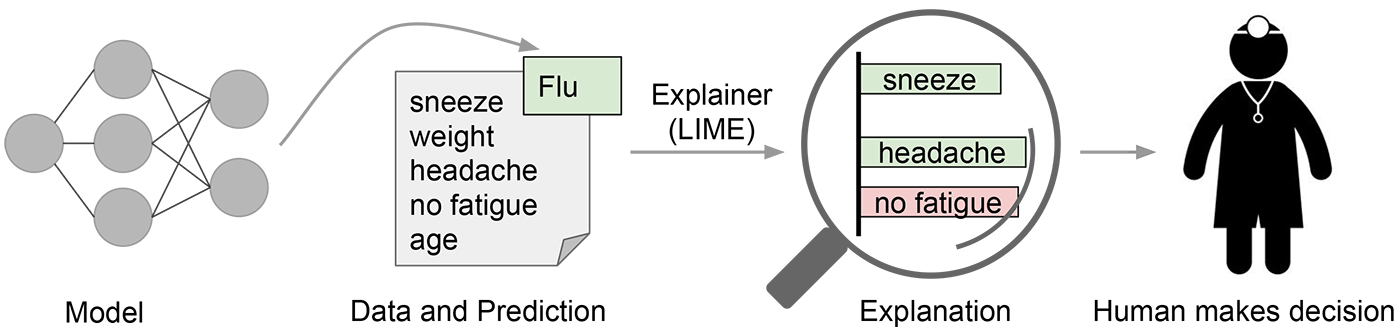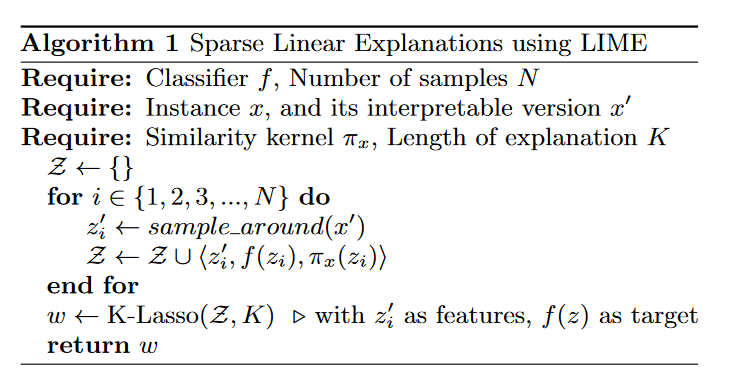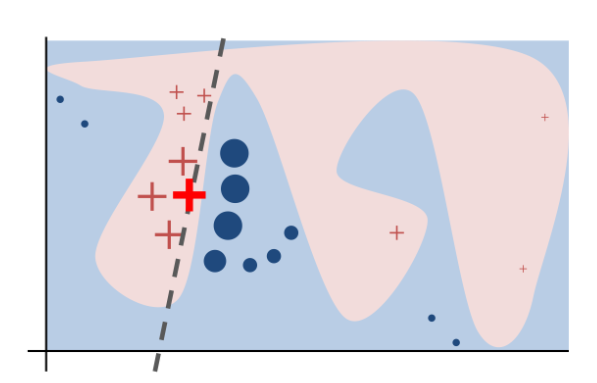# Explainable NLP with LIME

NLP models typically are black boxes in nature due to the large feature space stemming from the complexity of languages. However, explainable AI models seek to try to make clearer what the models are doing and how the classifiers in a given model work. This is especially important as key business personnel may not be willing to use a developed model if they don’t understand what it is doing. LIME specifically, is one such explainability model which utilizes perturbation to add and remove features to understand how each feature contributes to the final classification. This post will give an overview of LIME and how to use LIME to explain a simple classification problem using text data.## Local Interpretable Model-Agnostic Explanations(LIME)

Officially, the goal of LIME is to identify an interpretable model over the interpretable representation that is locally faithful to the classifier. We can look at the algorithm below:The goal is to get a vector which explains the lowest loss given the number of features we are using. The model will use lasso to minimize the number of features to match what we need. Features that are closer to our predicted value will have higher weights and vice versa based on our new linear decision boundary for this explaination. We can also visually see what is happening below:In the figure above, we can see visually how LIME works. The blue and the pink outlines represent a complex decision boundary. The + and circles represent different classes in a binary classificiation problem. The dashed line is the best locally faithful decision boundary to explain the bold red +. This local linear model tries to minimize the loss given the distances of the features to the given item we are trying to approximate. The closer features are to the thing we are predicting, the higher weights they have.

### Example

In this example, we will use the sentiment labeled review dataset from UCI’s Machine Learning Repository. This is a relatively small balanced dataset of 1000 review strings from Amazon based on their sentiment class.

LIME is model agnostic but does require passing in probabilties to understand how influential a given feature is to the classification probabilties. We load, create training and test split, and transform our data using tf-idf.

``````# Very simple TF-IDF Vectorizer
vectorizer = sklearn.feature_extraction.text.TfidfVectorizer()
train_vectors = vectorizer.fit_transform(train_x['review'])
test_vectors = vectorizer.transform(test_x['review'])
``````

We then build a simple random forest classifier by passing in the TF-IDF vectors.

``````# Build a basic random forest model
rf = sklearn.ensemble.RandomForestClassifier()
rf.fit(train_vectors, train_y)
``````

We then load lime and create a pipeline to TF-IDF vectorize and random forest.

``````from lime import lime_text
from sklearn.pipeline import make_pipeline
c = make_pipeline(vectorizer, rf)
``````

We then create a LIME explainer for this binary classification problem.

``````from lime.lime_text import LimeTextExplainer
explainer = LimeTextExplainer(class_names=[0,1])
``````

We then define a function to return the review and relevant probabilties changes from LIME.

``````def get_lime_probabilities(pipeline, explainer, index):
""" Get general explaination for a given review and prediction """
exp = explainer.explain_instance(test_x.review.iloc[index], pipeline.predict_proba, num_features=10)
print('Document id: %d' % index)
print('Review: %s' % test_x.review.iloc[index])
print('Probability matrix =', pipeline.predict_proba([test_x.review.iloc[index]]))
print('Predicted class: %s' % np.argmax(pipeline.predict_proba([test_x.review.iloc[index]])))
print('True class: %s' % [test_x.sentiment.iloc[index]])
print('Explaination for predicted class ', np.argmax(pipeline.predict_proba([test_x.review.iloc[index]])))
print(exp.as_list())
``````

Then we run the function to understand what is happening with this prediction.

``````get_lime_probabilities(c, explainer, 188)
``````
``````Document id: 188
Review: Also difficult to put on.I'd recommend avoiding this product.
Probability matrix = [0.47 0.53]
Predicted class: 1
True class: 0
Explaination for predicted class  1
[('recommend', 0.19046906785985251), ('difficult', -0.09131081603859173), ('this', 0.07592268719207586), ('put', -0.06069037154365428), ('product', -0.05550613449316974), ('Also', 0.04274818143763294), ('to', -0.024863375511585874), ('on', -0.0149428292025695), ('avoiding', -0.003307595401415095), ('I', -0.0014129873851774172)]
``````

We can see that this model is very confused about this prediction with almost 50/50 in classification. Looking at the text features, it is clear why. We have some weak features like `Also` but we have very strong features which influenced the decision boundary. In particular, `recommend` pushed the decision boundary to positive causing this misclassification.

As we can see, LIME is one approach to explain machine learning models. The algorithm is reasonable and works quickly as it searchs the local decision space rather than the global.